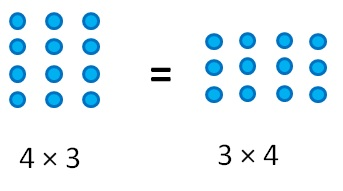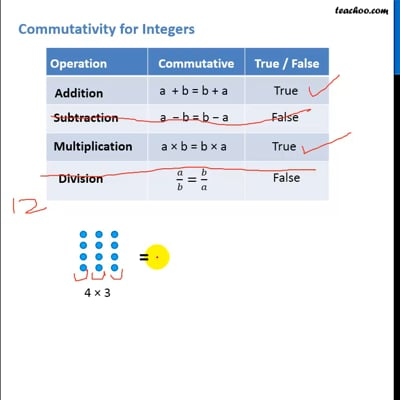Properties of Integers

Chapter 1 Class 7 Integers
Concept wise
 Operation Commutative True / False Addition a  + b = b + a True Subtraction a  − b = b − a False Multiplication a × b = b × a True Division a/b=b/a FalseSo commutativity is always possible for addition &

multiplication, but not for subtraction & division.

For Integers

Let us take two integers 2 & 3

 Operation Number Remark Addition a + b = b + a Take a = 2 & b = 3   L.H.S a + b = 2 + 3 = 5   R.H.S b + a = 3 + 2 = 5   ∴ a + b = b + a Since a + b = b + a, ∴ Addition is commutative. Subtraction a − b = b − a Take a = 2 & b = 3   L.H.S a − b = 2 − 3 = −1   R.H.S b – a = 3 − 2 = 1 ∴ a − b ≠ b – a Since a − b ≠ b − a, ∴ Subtraction is not commutative . Multiplication a ×  b =  b × a Take a = 2 & b = 3   L.H.S a × b = 2 × 3 = 6   R.H.S b × a = 3 × 2 = 6   ∴ a × b = b × a Since, a × b = b × a ∴ Multiplication is commutative. Division a/b=b/a Take a = 2, b = 3   L.H.S  a/b = 2/3   R.H.S  b/a = 3/2   ∴ a/b≠b/a Since a/b≠b/a  ∴ Division is not commutative .This video is only available for Teachoo black users